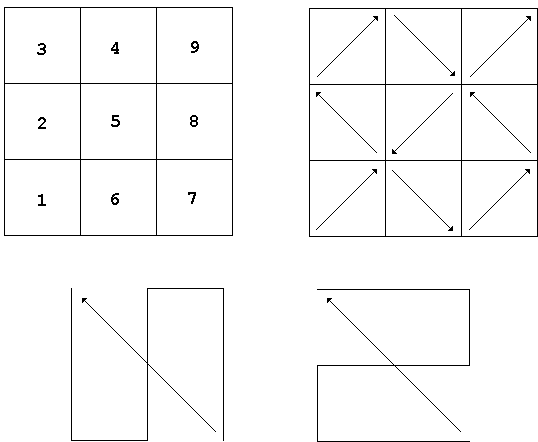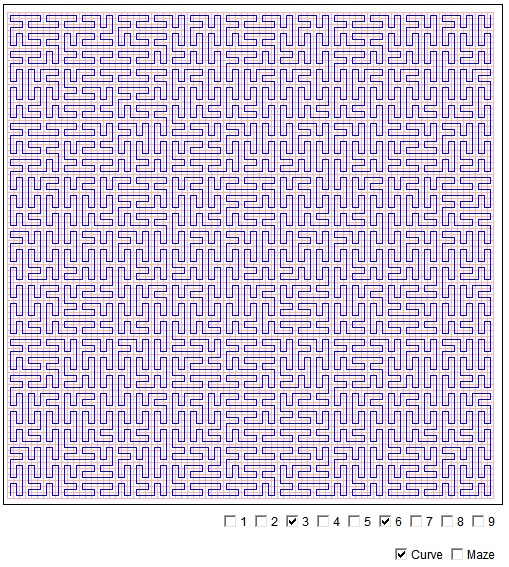Plane Filling Curves: All Peano Curves

The second step of Peano curve construction, when the unit square is split into 9 small squares, is the most crucial, because it defines the whole process. The pieces in two squares related to each other in the same manner regardless of the step of the construction. For example, if on the second step, a piece in square #3 is obtained from that in square #6 by means of reflection in x axis, the same will be true for pieces in squares 3 and 6 on every step of the construction.

The nine squares are ordered as shown below with the direction of traversal for each. For each of the four possible directions there are two ways to get from one corner to the opposite one in an "S" motion. The example shows the two possibilities for the traversal from the lower right corner to the upper left one.In all, there are 272 distinct curves.

As of 2018, Java plugins are not supported by any browsers (find out more). This Wolfram Demonstration, Peano Curve Explorer, shows an item of the same or similar topic, but is different from the original Java applet, named 'PeanoComplete'. The originally given instructions may no longer correspond precisely.

(image below from deprecated 'PeanoComplete' applet)Reference

1. H. Sagan, Space-Filling Curves, Springer-Verlag, 1994Plane Filling Curves•